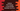# Python program to find change in percentage between two numbers## Python example program to find the change in percentage between two numbers :

In this post, I will show you how we can find the change in percentage between two numbers in python.

This is a beginner-friendly python program and you will get the idea of how we can take user inputs and how to calculate the difference in percentage in Python.

### Steps we will use in the program :

Following steps we will use in this program:

• Ask the user to enter the first number
• Ask the user to enter the second number
• The second number should be greater than the first number. Because we are finding the percent increase of the second number as compared to the first number. If this condition is false, enter one message that the numbers are invalid.
• Calculate the percentage difference between the two numbers.
• Print out the result

### Python program :

Let’s write down the python program :

``````first_number = int(input("Enter your first number :"))
second_number = int(input("Enter your second number :"))

if second_number < first_number:
else:
percent_diff = ((second_number - first_number)/first_number) * 100

print("Difference in percentage is : {}%".format(percent_diff))``````

### Explanation :

In this program :

• first_number is the first number that we are taking as input from the user.
• second_number is the second number. input() method is used to take input in console. We are converting the input to int using int(input()).
• The percent difference is calculated and stored in percent_diff variable.
• Finally, we are printing the calculated value, i.e. percent_diff

### Sample output :

``````Enter your first number :10